Critical Depth in Circular Pipe (Culvert) Calculation

Critical depth is a function of the flow rate and pipe diameter

Register to enable "Calculate" button.

 Diameter, D: inch ft cm m Flowrate, Q: gal/min (gpm) gal/day (gpd) Million gal/day (MGD) ft3/s (cfs) m3/s m3/day Critical Depth, Yc: inch www.LMNOeng.com © 2013 LMNO Engineering, Research, and Software, Ltd.

Units in critical depth calculation: cm=centimeter, ft=feet, gal=US gallons, m=meters, min=minute, s=second

Introduction
For water flowing in a culvert, it is important to know the critical depth. Though water may not actually be flowing at the critical depth, it is helpful to know if the actual water depth is greater than or less than critical. Critical depth is a quantity of fundamental importance to understanding the flow characteristics. If the actual depth is greater than critical depth, then the flow is considered "subcritical". Subcritical flow is "slow flow" and is impacted by downstream conditions. If the actual depth is less than critical depth, then the flow is "supercritical". Supercritical flow is "fast flow" and is impacted by upstream conditions. It flows faster than the wave speed and is unimpacted by downstream conditions. A detailed discussion of subcritical and supercritical flow can be found on our gradually varied flow calculation page.

As water flows down a culvert, the depth may change and pass through the critical depth if the bottom slope, geometry, or pipe material changes. If the flow is initially subcritical and the channel slope increases, the water may undergo a hydraulic drop if it transitions from subcritical to supercritical. Conversely, if the flow is supercritical and the culvert slope becomes flatter, then there could be a hydraulic jump such that the water passes through the critical depth as it becomes subcritical.

Equations
For a circular pipe, critical depth can be computed based on first principles (mass, momentum, and energy conservation). For a mild downward sloping culvert (slope less than about 10%), the specific energy is (Chow, 1959, p. 41):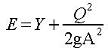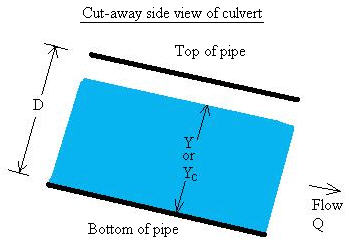The channel cross-sectional area can be written in terms of the geometry as (Chow, 1959, p. 21):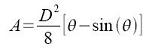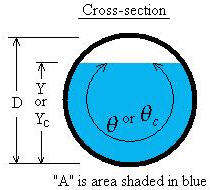Critical depth occurs when energy is at a minimum with respect to depth, dE/dY=0. The following equation is obtained when energy is minimized: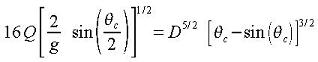LMNO Engineering uses a numerical solution method to solve the above equation for θc and then for Yc.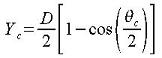Notation
Our calculation allows you to use a variety of units with all of the conversions computed internally. The units shown below are SI (international system of units).
A = Flow area of water, m2.
D = Inside diameter of pipe (culvert), m.
E = Specific energy, m.
g = Acceleration of gravity = 9.8066 m/s2.
Q = Flow rate (discharge), m3/s.
V = Velocity, m/s.
Y = Water depth, m.
Yc = Critical depth, m.
θ = Angle of water in cross-section, radians.
θc = Angle at critical depth, radians.

Messages given by calculation
"Need Diameter > 0", "Need Flowrate > 0", "Need D≥1e-6 m", "Need D≤1000 m", "Need Q≥1e-9 m3/s", "Need Q≤1e6 m3/s". These are input checks. Input diameter and flow rate must be positive numbers. D must be between 10-6 m and 1000 m. Q must be between 10-9 m3/s and 106 m3/s.

"Need larger Q or smaller D". Run-time error. The combination of flow rate and diameter that were input result in a very small critical depth approaching machine precision.

"Need smaller Q or larger D". Run-time error. The combination of flow rate and diameter that were input result in critical depth greater than the pipe diameter.

Reference
Chow, V. T. 1959. Open-Channel Hydraulics. McGraw-Hill, Inc.

LMNO Engineering, Research, and Software, Ltd.
7860 Angel Ridge Rd.   Athens, Ohio  45701   USA   (740) 707-2614
LMNO@LMNOeng.com    http://www.LMNOeng.com

To:

Related calculations:

Manning Equation for Circular Culvert

Culvert Design using Inlet and Outlet Control

Flowrate using End Depth Method

Hydraulic Jump in a Pipe or Culvert

Unit Conversions Page

Register